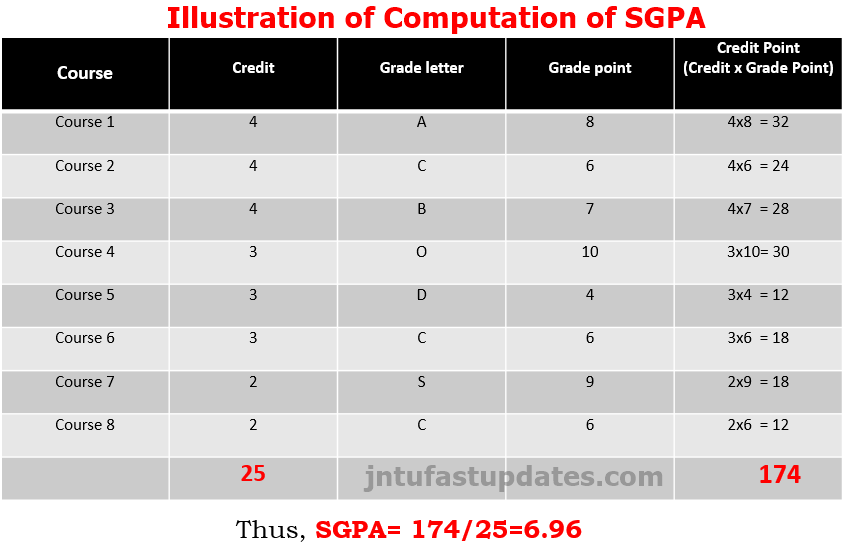# JNTUK How to Calculate SGPA & CGPA – JNTU Kakinada Computation of Grade Point Averagae

77

JNTUK How to Calculate SGPA & CGPA –  how to Get Marks from Grades, JNTU Kakinada Computation of Semester Grade Point Averagae (SGPA) & Cumulative Grade Point Average (CGPA).## JNTUK How to Calculate SGPA & CGPA – JNTU Kakinada Grade Point Averagae

Letter Grades and Grade Points: The absolute grading mechanism is followed in mapping the letter grades. The marks are converted to grades based on pre-determined class interval. As per the UGC recommendations a 10-point grading system with the following letter grades are followed. The same is furnished in the following table.

 Grade Points R20 R19 R16 10 A+ O O 9 A S S 8 B A A 7 C B B 6 D C C 5 E D D 0 F F F

A student obtaining Grade F shall be considered failed and will be required to reappear in the examination. For non-credit courses ‘Satisfactory’ or “Unsatisfactory’ shall be indicated instead of the letter grade and this will not be counted for the computation of SGPA/CGPA.

## Semester Grade Point Average (SGPA) and Cumulative Grade Point Average (CGPA)

Grade Point: It is a numerical weight allotted to each letter grade on a 10-point scale.

Letter Grade: It is an index of the performance of students in a said course. Grades are denoted by letters O, S, A, B, C, D and F.

### JNTUK SGPA CalculatorHow to calculate sgpa in engineering: The following procedure is to be adopted to compute the Semester Grade Point Averagae. (SGPA) and Cumulative Grade Point Average (CGPA):

The SGPA is the ratio of sum of the product of the number of credits with the grade points scored by a student in all the courses taken by a student and the sum of the number of credits of all the courses undergone by a student, i.e

SGPA (Si) = Σ (Ci X Gi) / Σ Ci

Where Ci is the number of credits of the ith course and Gi is the grade point scored by the student in the ith course.

### Illustration of Computation of SGPA

How to calculate SGPA in percentage:### JNTUK Computation of CGPA

The CGPA is also calculated in the same manner taking into account all the courses undergone by a student over all the semester of a programme, i.e.

CGPA = Σ (Ci X Si) / Σ Ci

Where Si is the SGPA of the ith semester and Ci is the total number of credits in that semester.

### Illustration of Computation of CGPA

How to calculate SGPA to CGPA:• The SGPA and CGPA shall be rounded off to 2 decimal points and reported in the transcripts.
• While computing the GPA/CGPA the subjects in which the student is awarded Zero grade points will also be included.

### How to calculate percentage from CGPA

Conversion of grades into percentage: •Conversion formula for the conversion of GPA into Percentage is

[CGPA Earned – 0.75] x 10= Percentage of marks scored.

Illustration: [CGPA Earned: 8.18 – 0.75]x 10 = 74.3%

#### JNTUK R16 Class Awarded

After a student has satisfied the requirements prescribed for the completion of the program and is eligible for the award of B. Tech. Degree, he shall be placed in one of the following four classes:1.Muzahid
2.SUNDAR